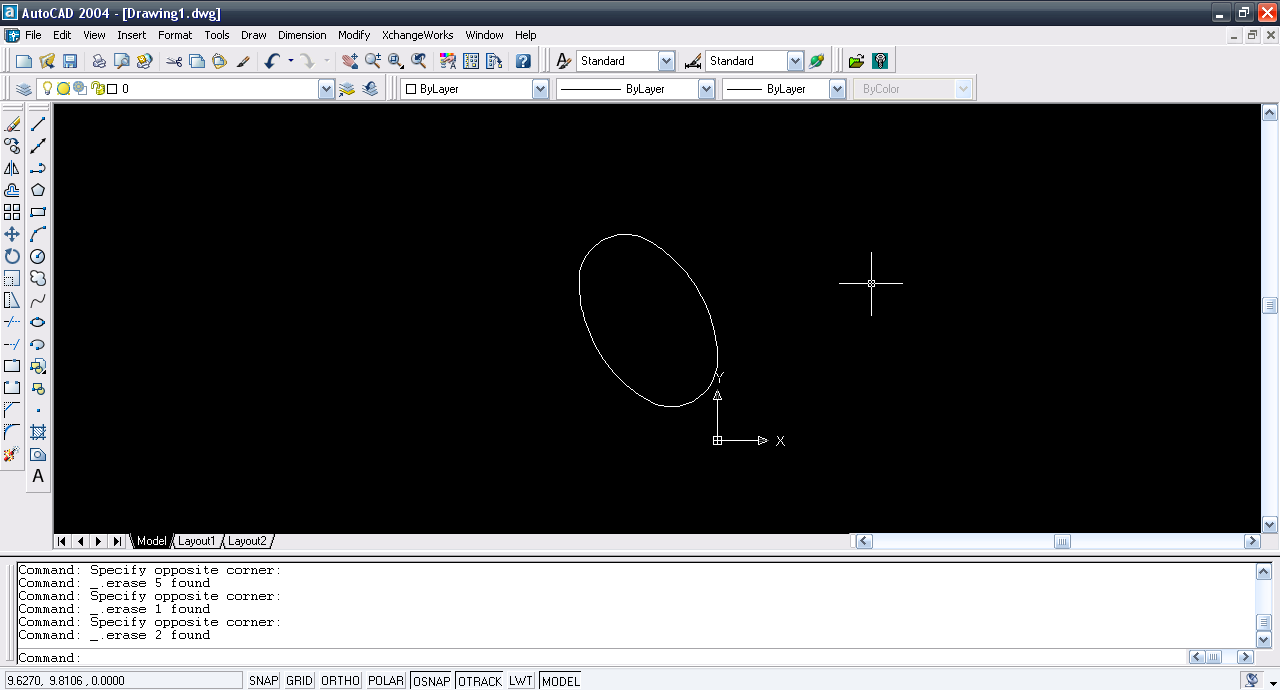# Tutorial - Creating isometric circle (Front view) in AutoCAD?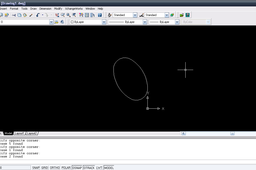Here is the tutorial.

1. ### Step 1: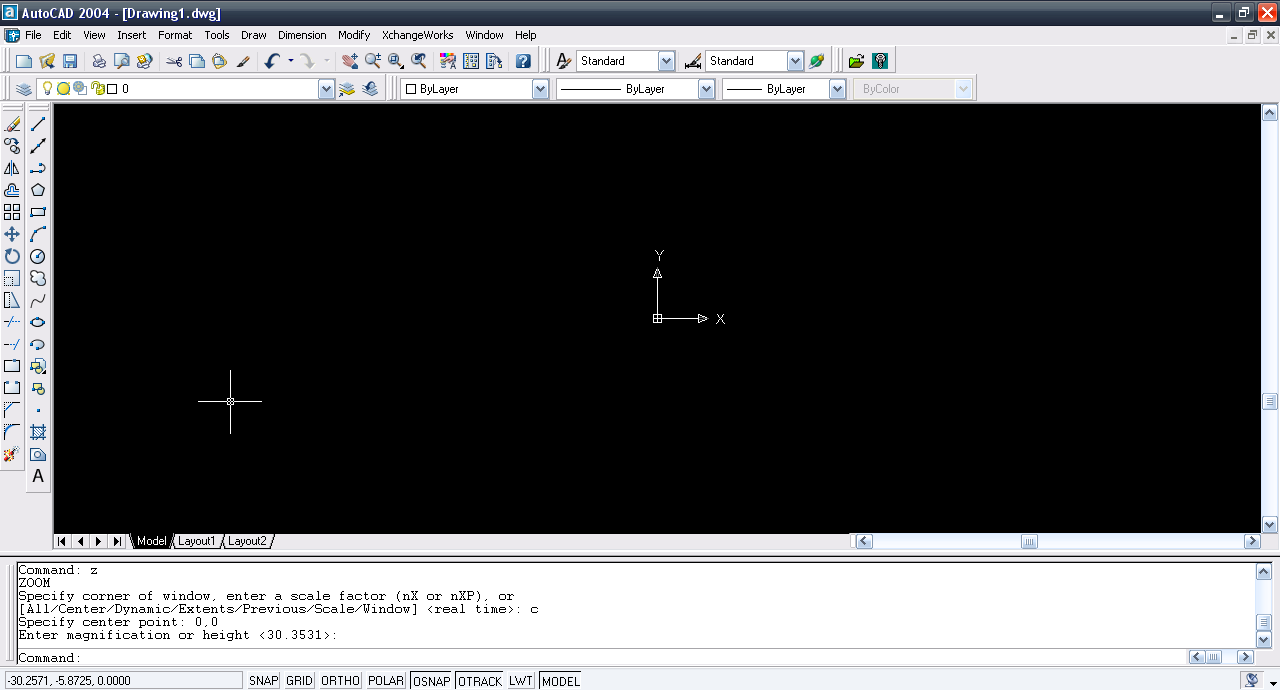2. ### Step 2:

Use line tool and input these values=
(0,0),
@10<270,
c.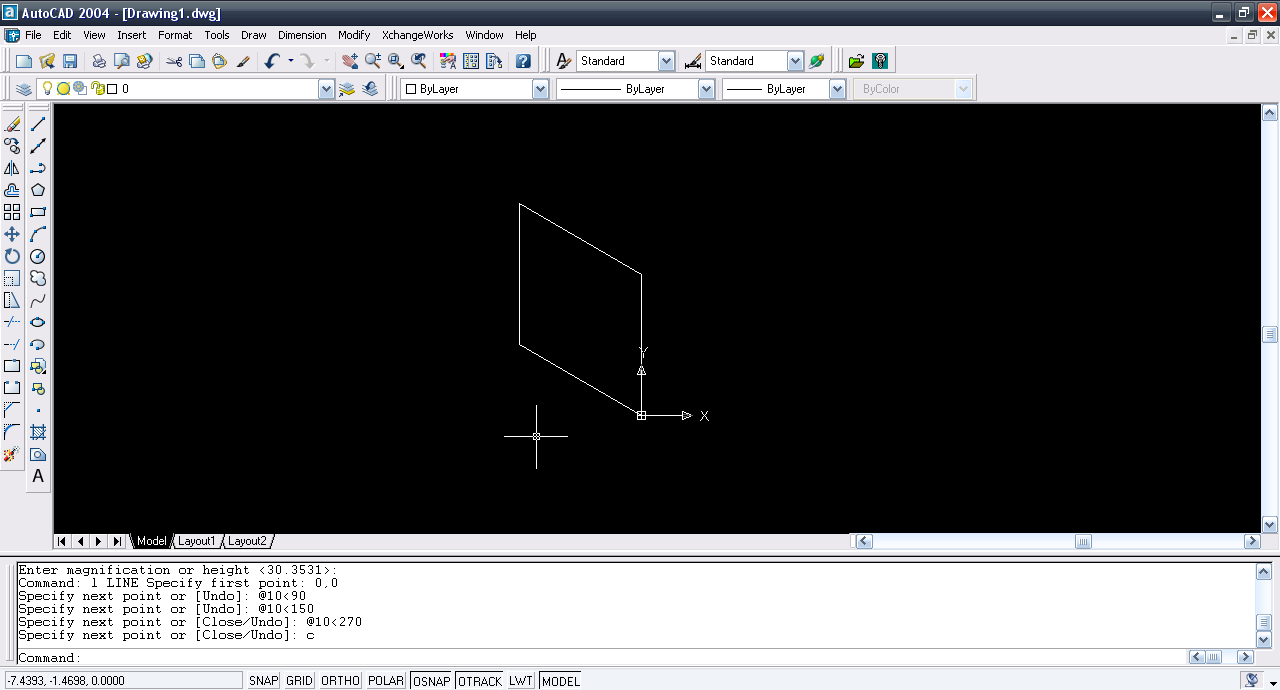3. ### Step 3:

Now draw lines to the mid-points of the the lines.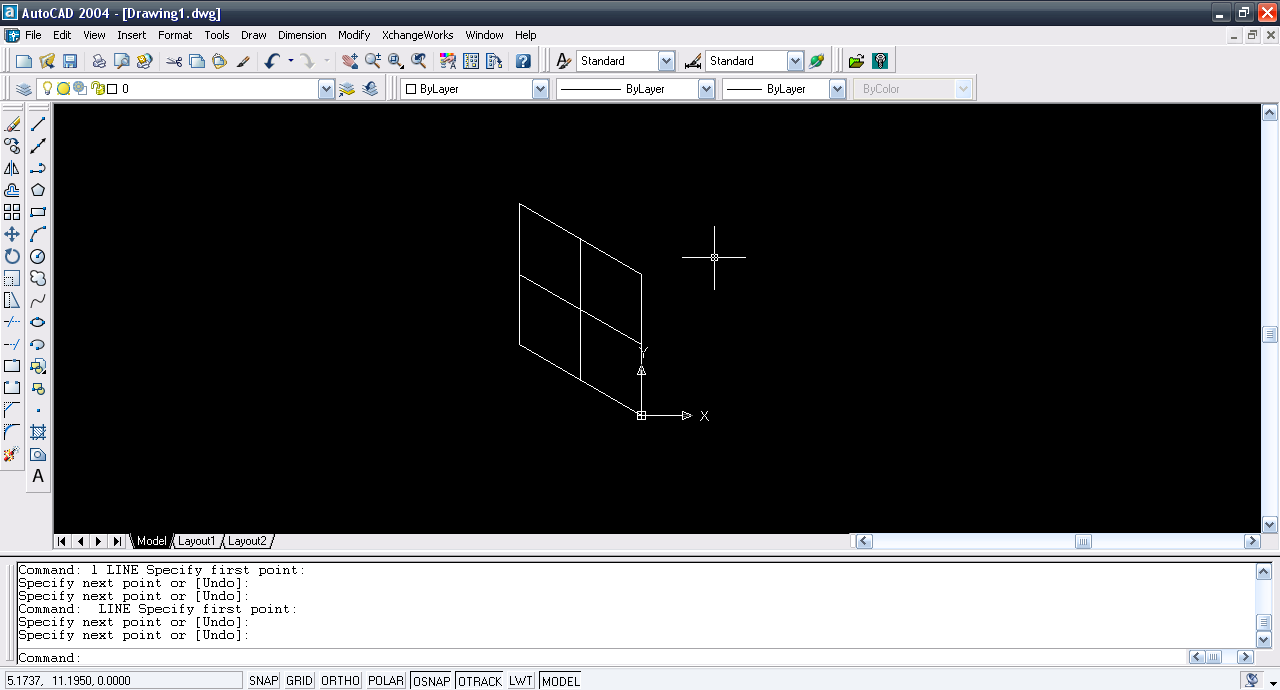4. ### Step 4:

Draw these lines.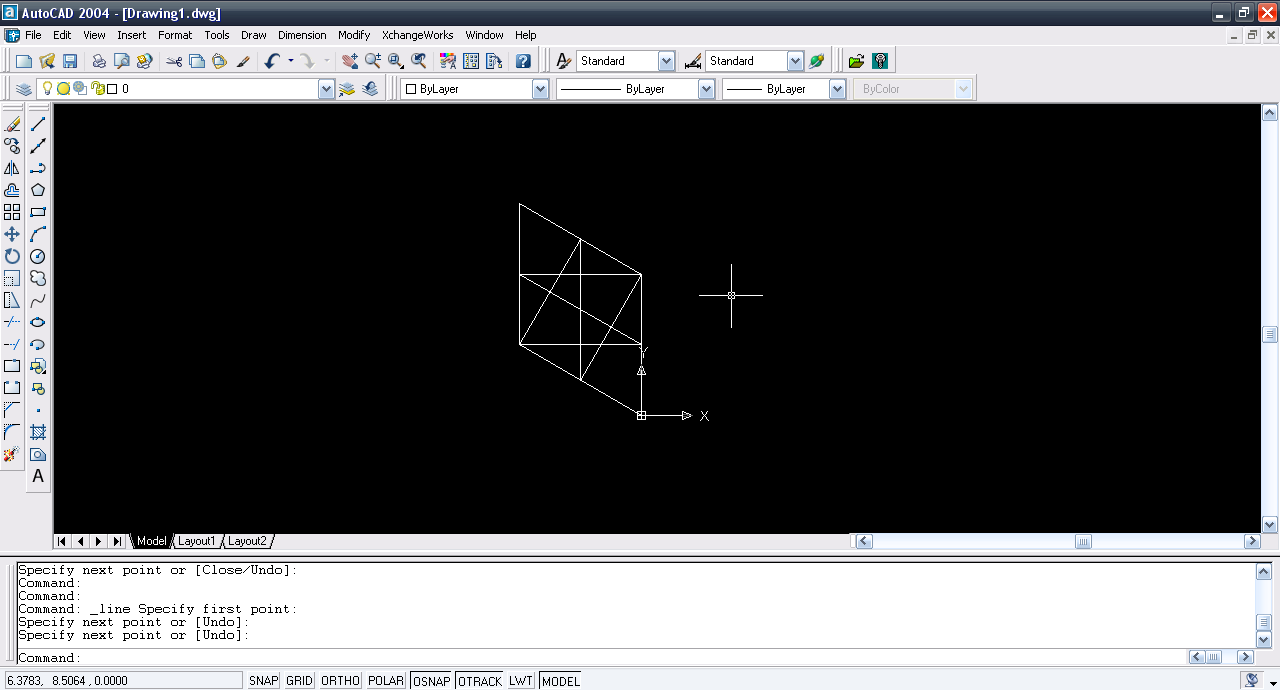5. ### Step 5:

Use circle tool and then select this intersection as center.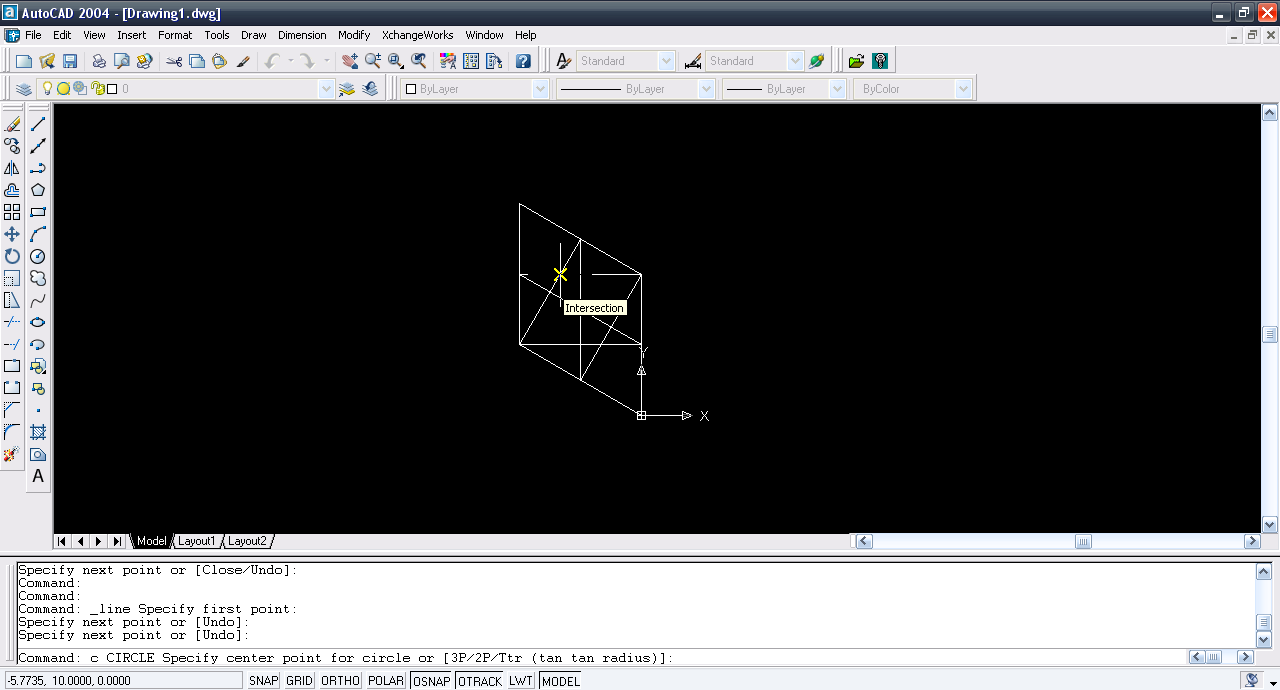6. ### Step 6:

Select the end point of the line.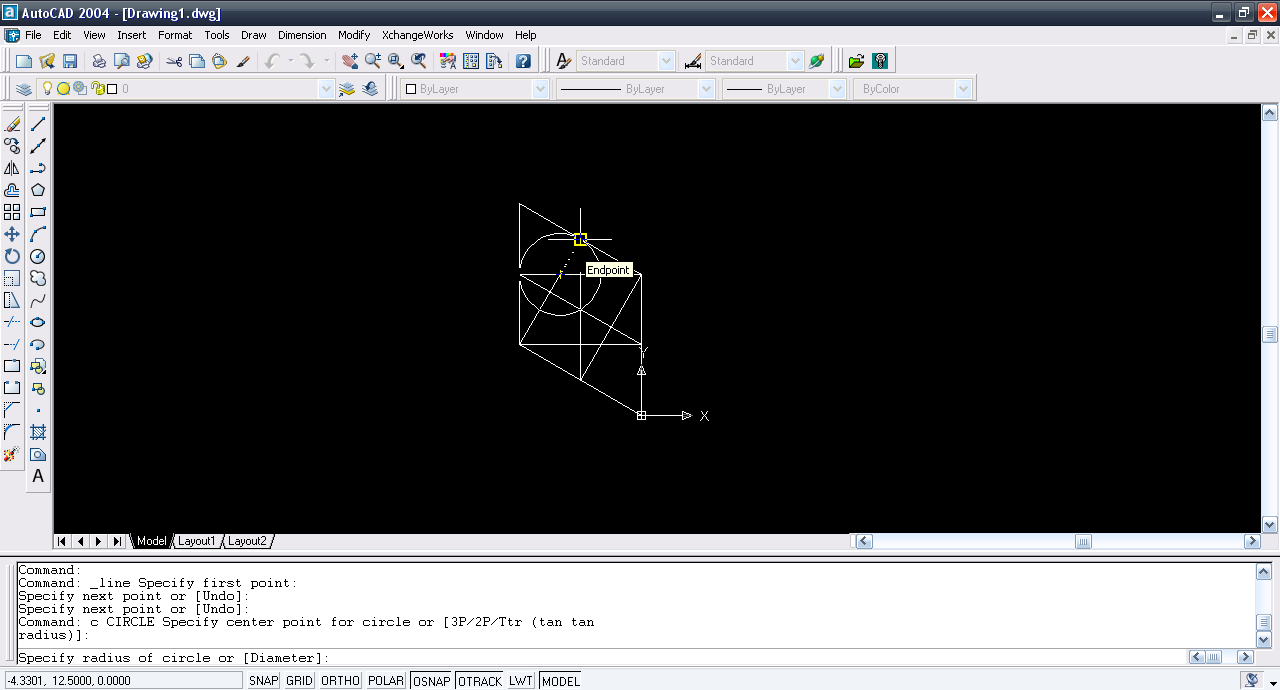7. ### Step 7:

Again circle tool and select the intersection.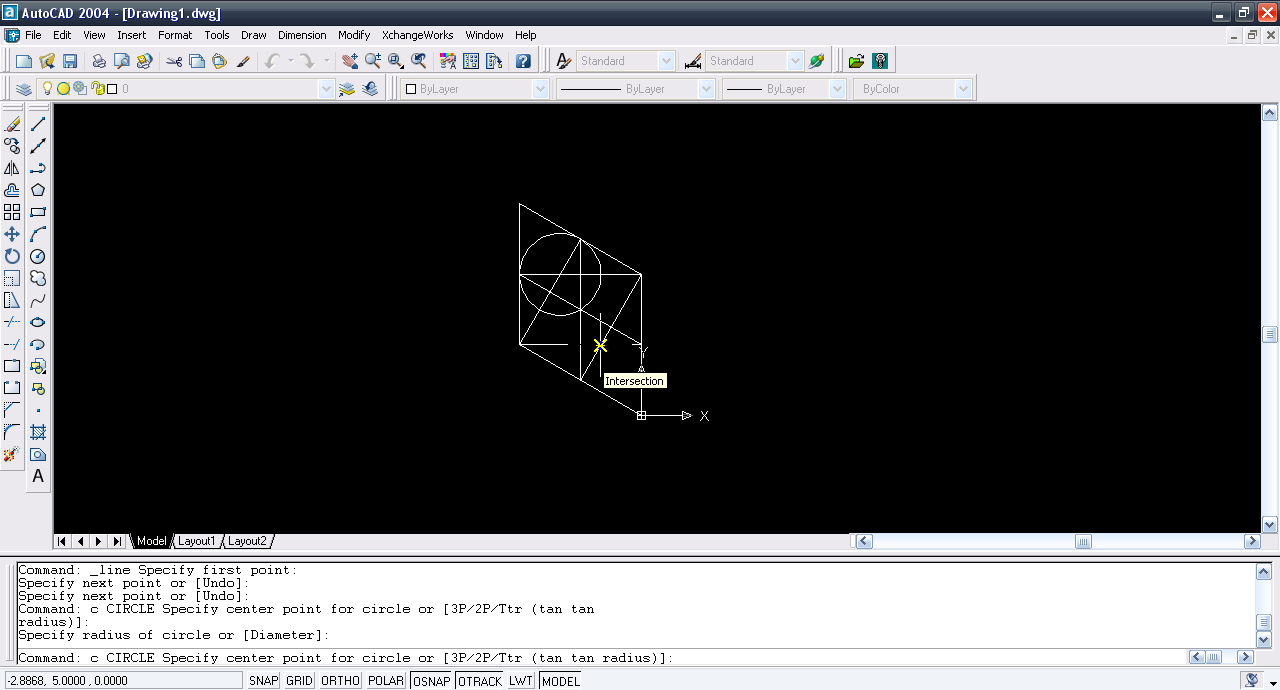8. ### Step 8:

To the end point of the line.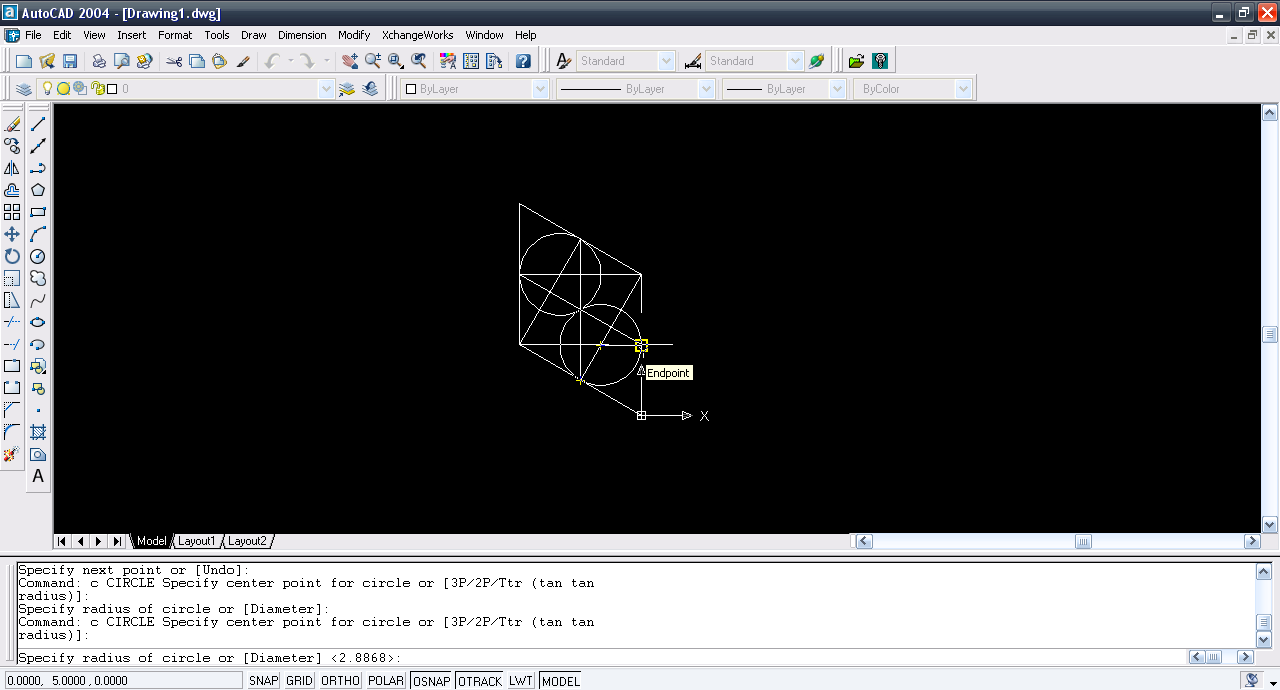9. ### Step 9:

Select circle tool and select the corner.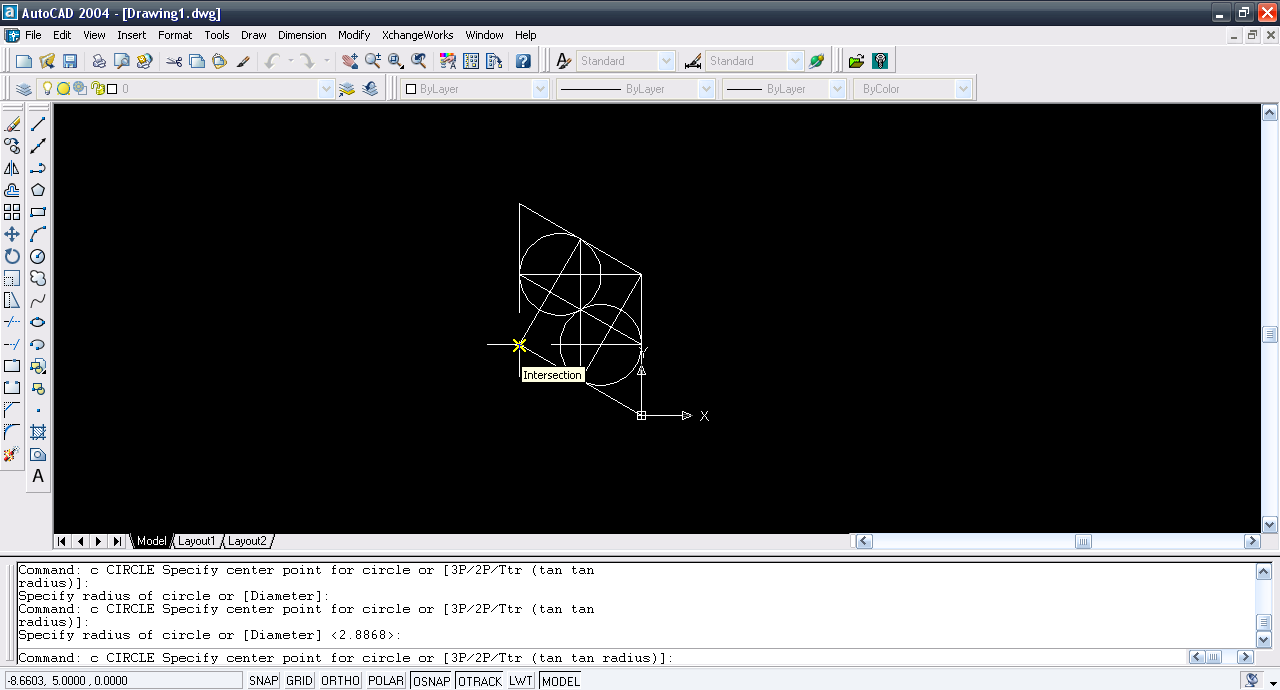10. ### Step 10:

To the mid-point of the upper line.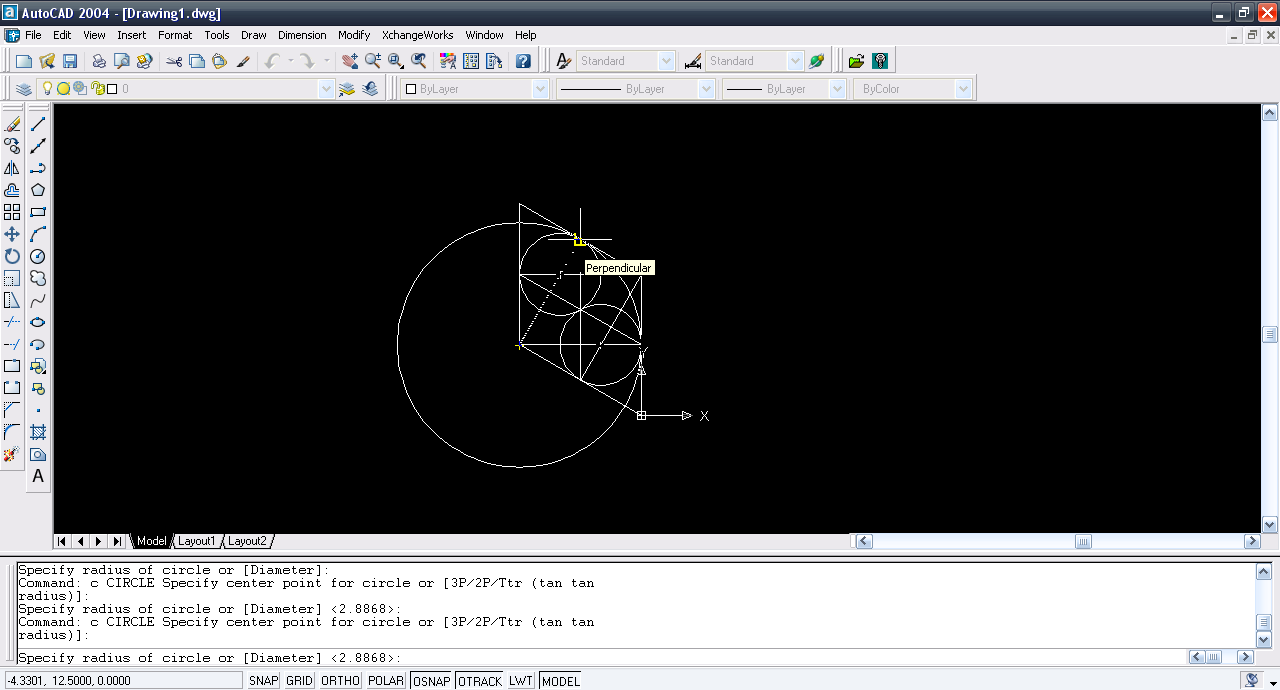11. ### Step 11:

Again circle tool and end-point.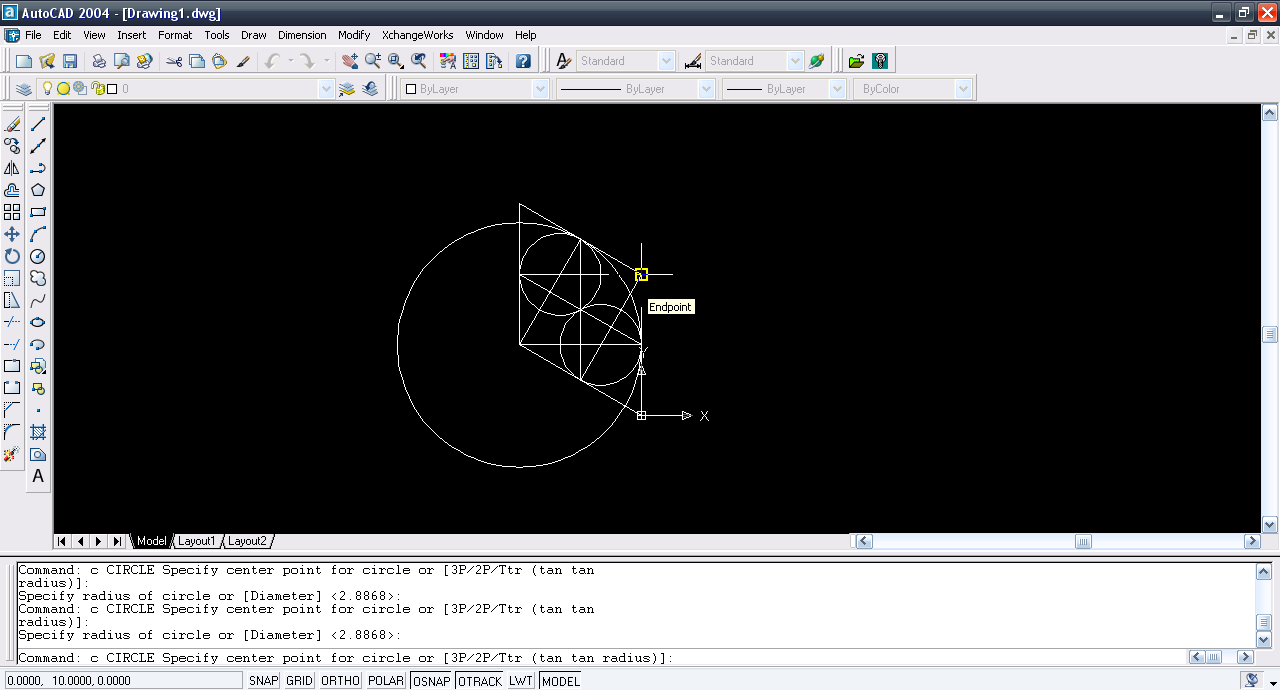12. ### Step 12:

To the other end-point.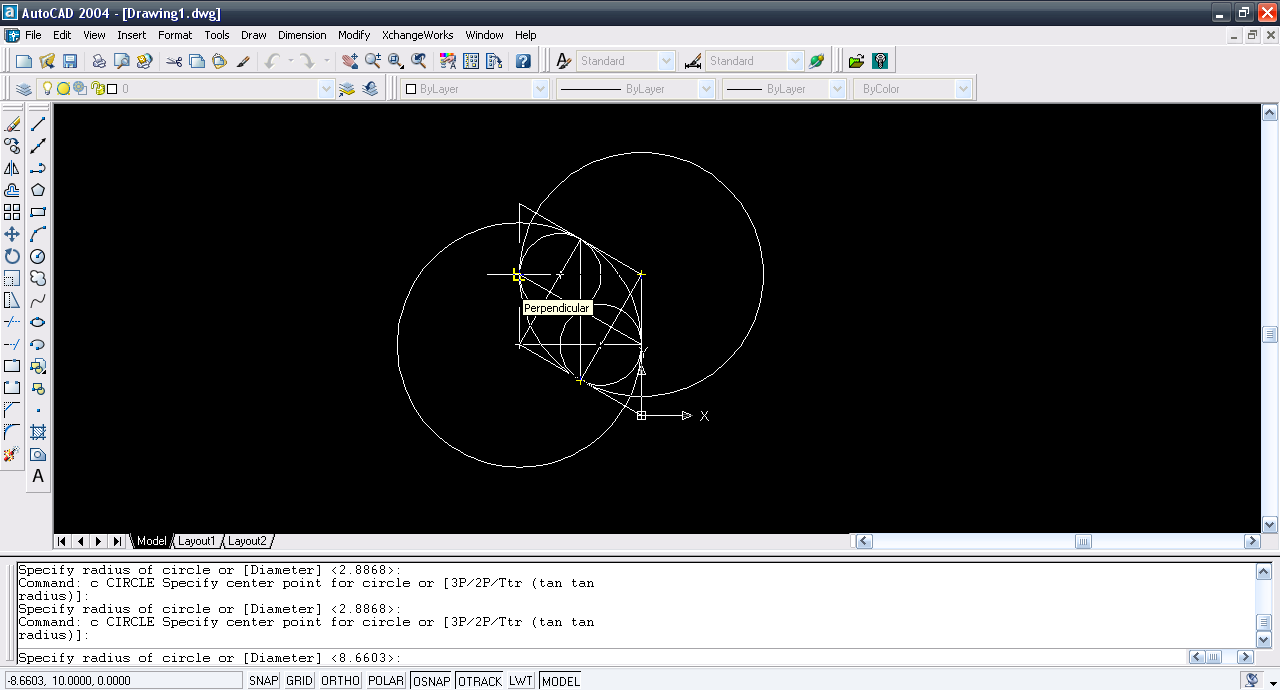13. ### Step 13:

Trim tool and select all.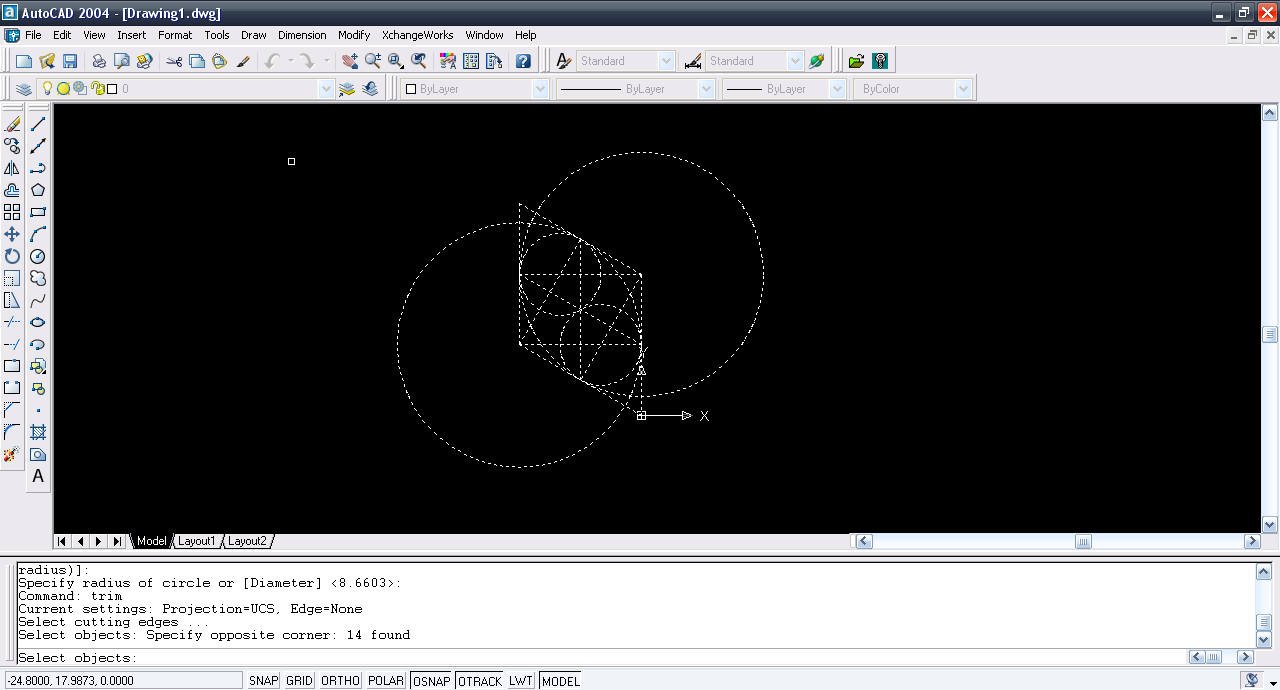14. ### Step 14:

Trim away the components leaving the circles to arcs.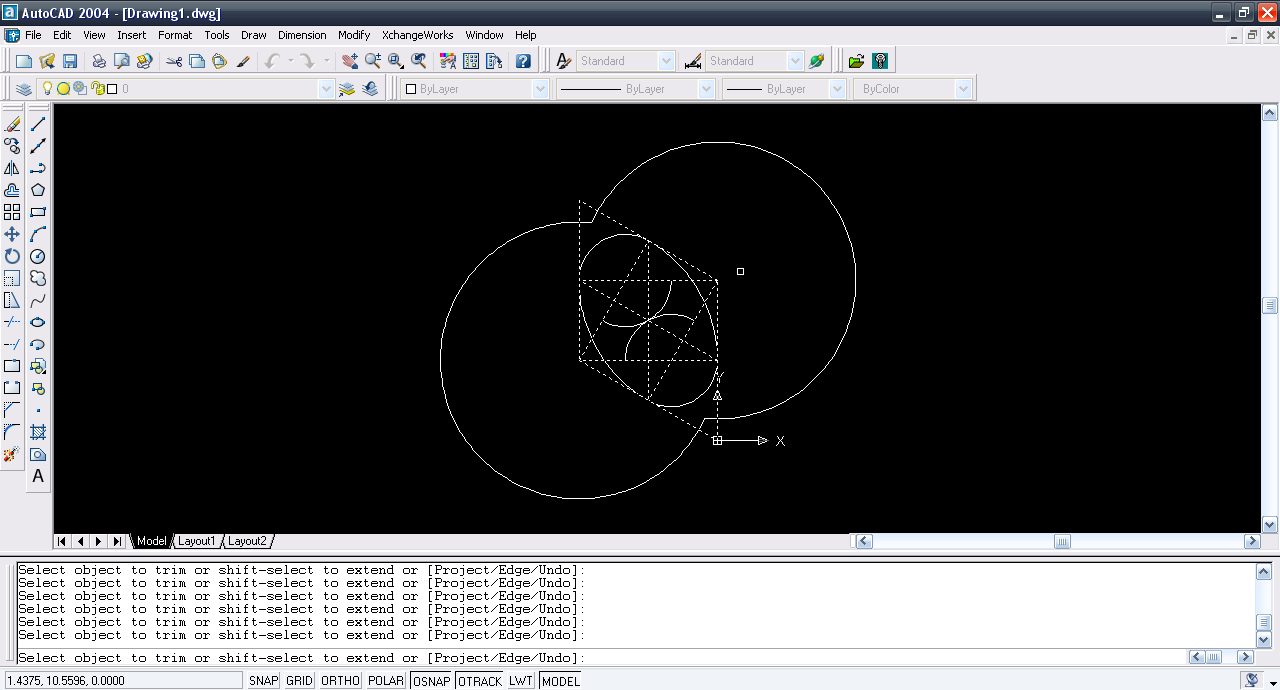15. ### Step 15:

Delete all construction and we have circle.